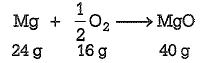1 g of magnesium is burnt with 0.56g of oxygen in a closed vessel. Which reactant is left in excess and how much?

(At. weight of Mg = 24, O=16)

(a) Mg, 0.16g

(b) O2, 0.16g

(c) Mg, 0.44g

(d) O2, 0.28g

Concept Videos :-

#5 | Stoichiometry
#6 | Questions on POAC
#13 | Calculation of % composition & % Purity

Concept Questions :-

Equation Based Problem

(a) The balanced chemical equation isFrom the above equation, it is clear that, 24g of Mg reacts with 16g of O2 .

Thus,1.0 g of Mg reacts with 16/24g of O2  = 0.67g of O2

But only 0.56g of O2 is available which is less than 0.67g. Thus,O2 is the limiting reagent. Further, 16g of O2 reacts with 24g of Mg.

0.56g of O2 will react with Mg = 24/16x0.56 = 0.84g

Amount of Mg left unreacted = (1.0-0.84)g Mg = 0.16g of Mg

Difficulty Level:

• 50%
• 20%
• 25%
• 8%